## Changing the Scale: Transforming Data

Started ‎06-15-2020 by
Modified ‎06-15-2020 by
Views 2,406

## Why Transform Data?

In this article I’ll discuss five reasons we might use a function to change the scale or “transform” data: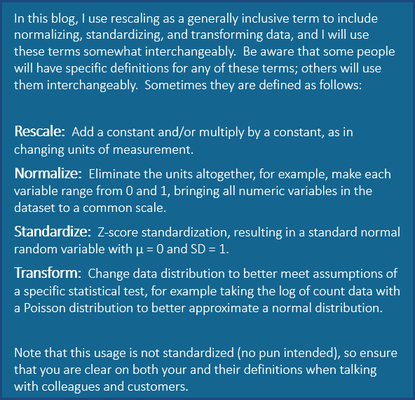Select any image to see a larger version.Mobile users: To view the images, select the "Full" version at the bottom of the page.
1. Collaboration
2. Meeting model assumptions
3. Activation functions and pooling in neural networks
4. Interpretability and appearance
5. Comparability

Note that these transformations may occur in preprocessing, as part of a modeling algorithm, or when postprocessing the data.

1. Collaboration

Musicians playing together must all be playing in the same key. If a band gets a new singer who sings in a different range, the band can adjust the scale up or down for her. For example, the musicians might change from key of F# to the key of C to accommodate the new singer’s range. Likewise, you might change medical data on patient temperature from Fahrenheit to Celsius to collaborate in an international study.

2. Meet Model Assumptions

Many variables do not meet the assumptions of parametric statistical tests. For example assumptions of linear models include:

• Normal (Gaussian) distribution of the residuals
• Homoscedasticity (equal variance)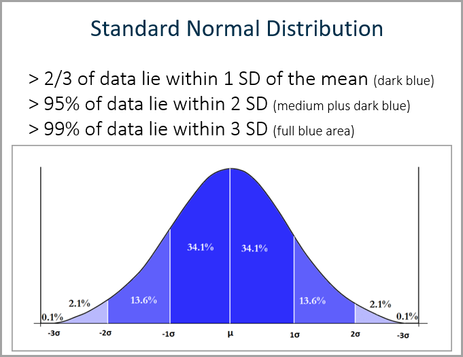Lack of normality affects standard errors and may also affect parameter estimates. Heteroscedasticity (variance that is not constant) compromises the standard errors. Using a parametric statistical test (such as a t-test, ANOVA, or linear regression) on these data can give false results.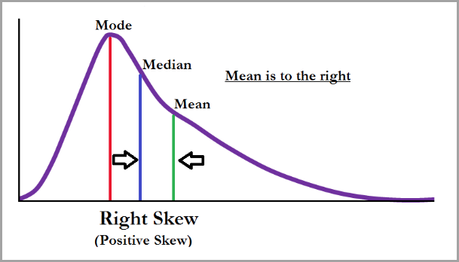But never fear! Maybe we will simply choose a different model. Alternatively, we can use transformations to change the shape of a distribution by replacing the variable with a function of that variable. Often, we can transform the data that are skewed (asymmetric) or non-linear to make them better comply with the assumptions of our statistical tests. In fact sometimes a single transformation will solve multiple problems:  reduce skew, get you closer to equal variance, and produce a nearly linear or additive relationship.

See below the histograms of home values from the HMEQ data set. VALUE is on the horizontal axis, and frequency is on the vertical access. We see that in the original data (IMP_VALUE) this variable distribution is skewed right. That is, there is a piling up of data at the mid to lower values, and a long tail to the right. However, after we use Enterprise Miner’s Transformation Node to take a log transformation (LOG_IMP_VALUE), the histogram more closely resembles a bell-shaped curve, i.e., a normal (Gaussian) distribution.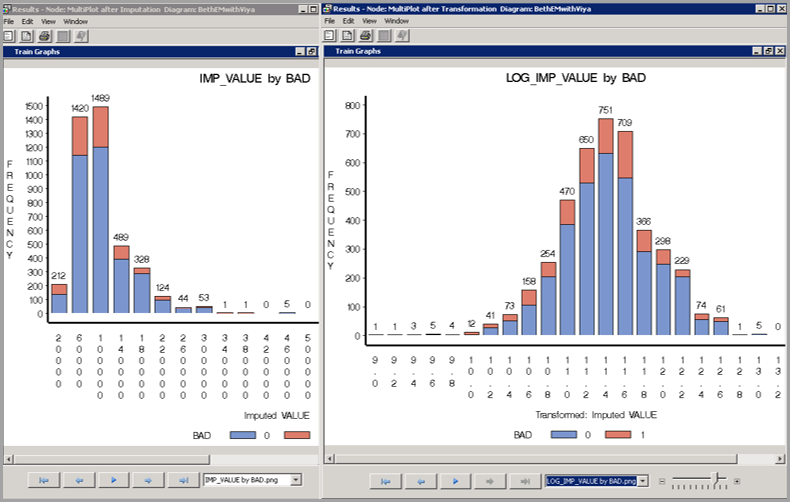Common transformations include: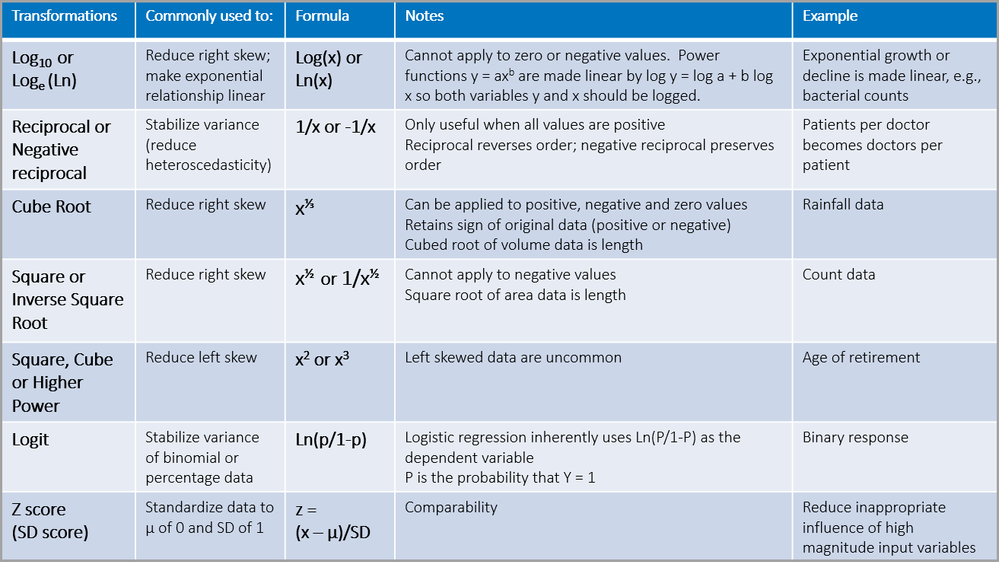Note:  You may have heard of the arcsine transformation. Arcsine transformations were used in the past for binomial data to be analyzed with linear regression, but this practice has fallen out of favor. Instead of transforming the data you can simply use logistic regression for binomial data.

3. Activation Functions for Neural Networks

Activation functions occur in the hidden layers in neural networks.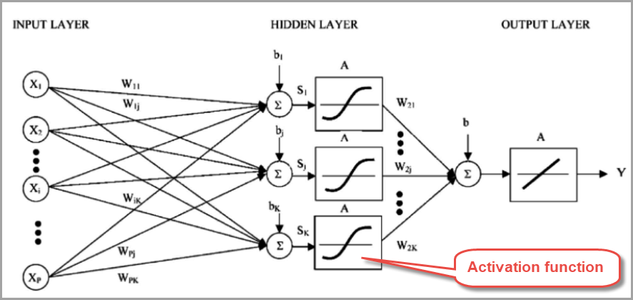They can “squish” a value into a desired range.  Commonly used functions are: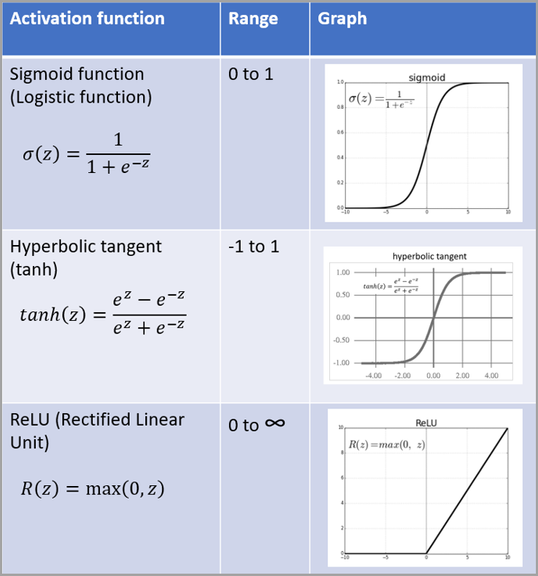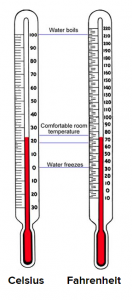4. Interpretability and Appearance

Use units appropriate to your field and audience. For example, if you are targeting American tv audiences with dashboards, you would display temperatures in Fahrenheit. For reporting, it can be helpful to back transform to the original units to make the results more understandable. Let's use the example of house values where the original units are dollars.  Rather than showing results (e.g., means and standard errors) as the log of house values, you would back transform (in this case take the antilog) to show results in the original unit of dollars.

5. Comparability

If your inputs to a model have vastly different magnitudes, it can help to standardize your data to make the input variables comparable in magnitude. For example, a variable like home value that ranges between 0 and 250 million will outweigh a variable like proportion of home used as office space, which ranges between 0 and 1. Using these variables as model inputs without normalization will give the variable with the larger range more weight in the analysis. Standardizing the data using a z score is one way to ensure that input variables are weighted equally.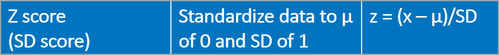In any variable transformations, consider what is customary or standard in your field. For example, FICO credit scores range from 300 to 850, so any final results for credit scoring must be standardized to this value.

Image classification commonly uses a softmax function to return final values between 0 and 1, as in the example shown below.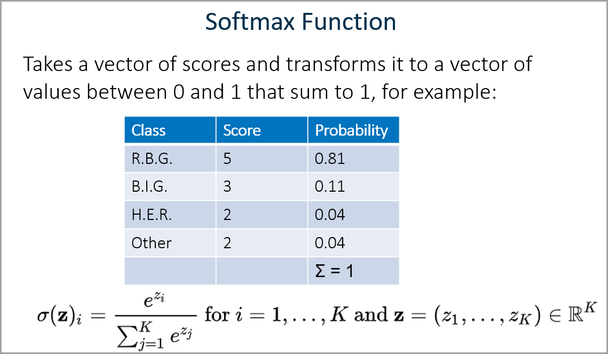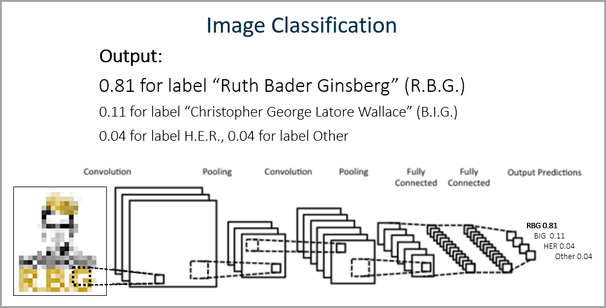For more about computer vision and convolutional neural networks, see my articles on:

### Example

Let’s look at vehicle accident fatalities in the United States during 2018 using SAS Visual Analytics 8.5. California, Texas, and Florida stand out as the states with the highest number of fatalities. Does this mean that driving is more dangerous in these states?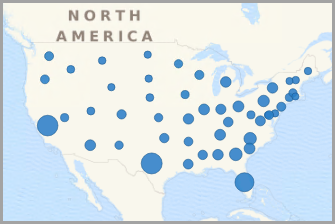We notice these three states also have very large populations.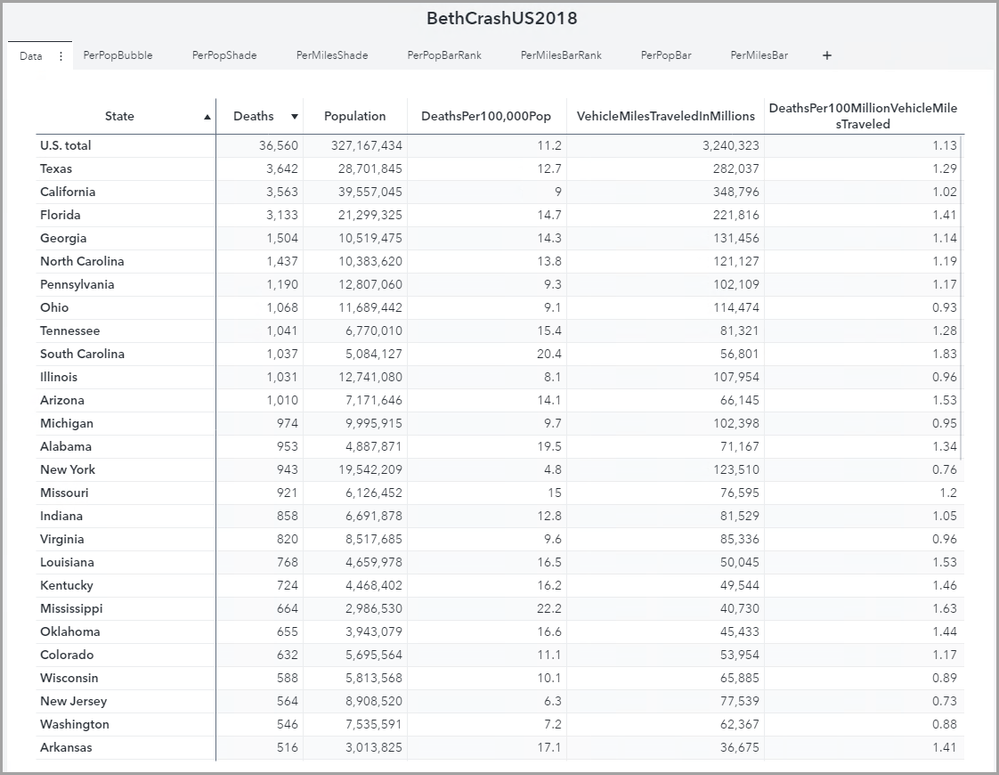Let’s take a second look. This time, let’s look at deaths per 100,000 people. This is illustrated in the map on the right below. Now we can more reasonably compare states to each other.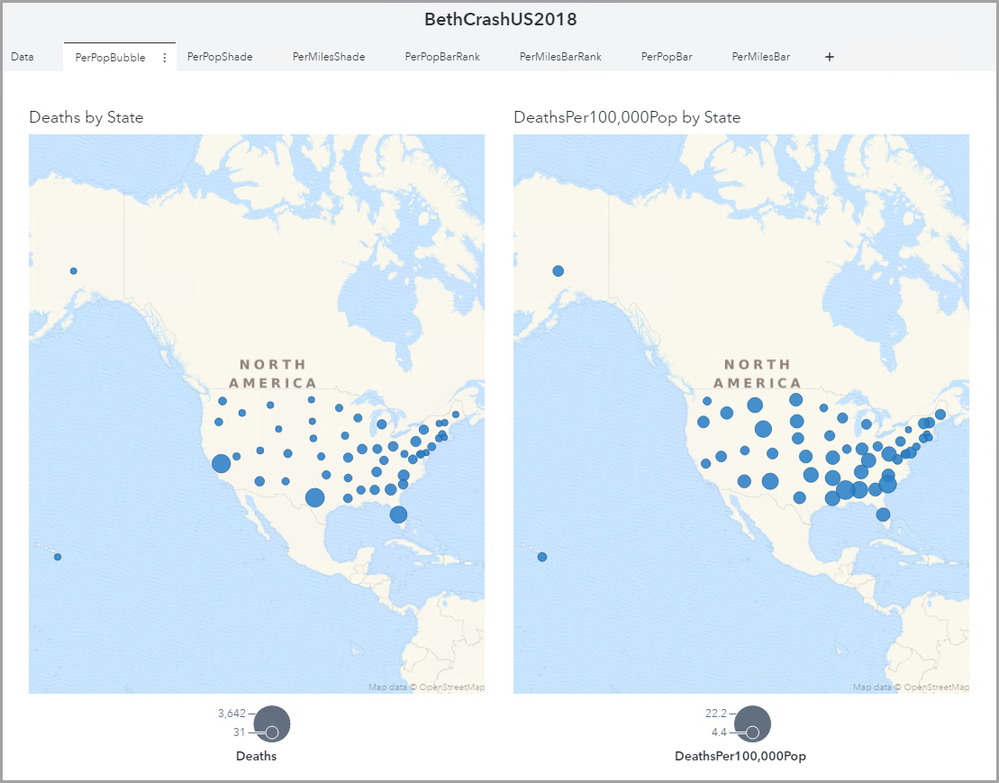We now see that California, for example, has a small bubble compared to, say, New Mexico.

If we prefer, we can see this same information with color in a geographic region map, as shown below. Again we have total deaths on the left, and we have deaths per 100,000 people in the map on the right. We see the darkest blue, or highest death rate, is in Mississippi.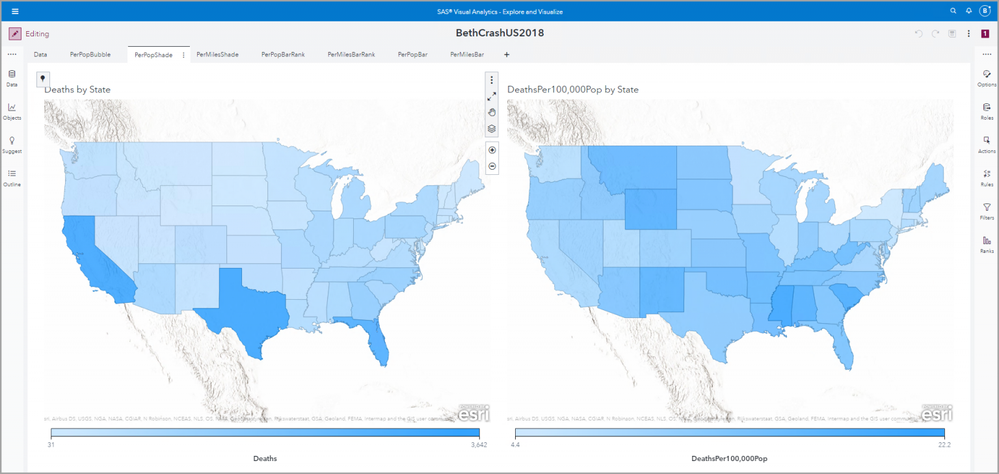We can look at this same information in a bar chart. The purple bars are death numbers. The green bars are deaths per 100,000 population. Again we see that although Texas has the highest number of vehicle accident deaths, Mississippi has the highest vehicle accident death rate per 100,000 people.A common way to look at fatality rates in the transportation field is to look at the number of deaths per million miles traveled. We see this below in the map on the right.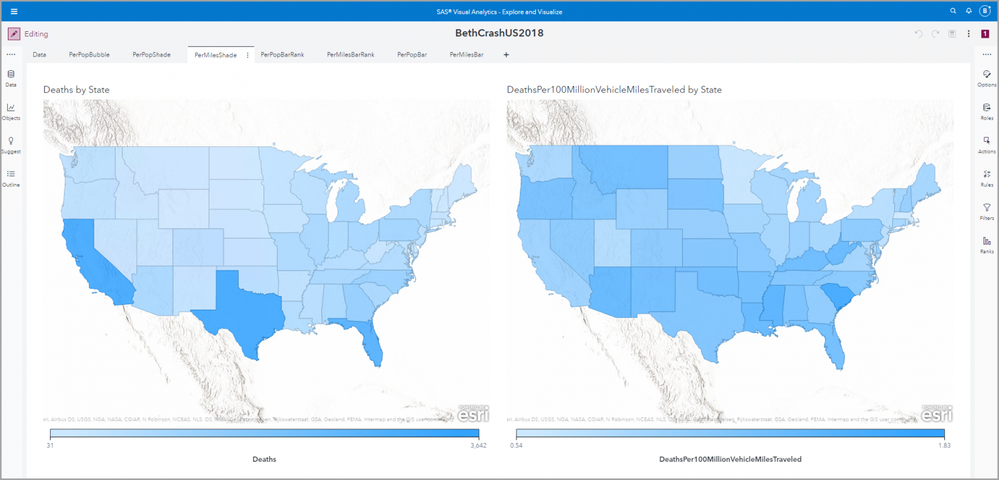Here we see deaths in purple and death per 100 million vehicle miles traveled in orange.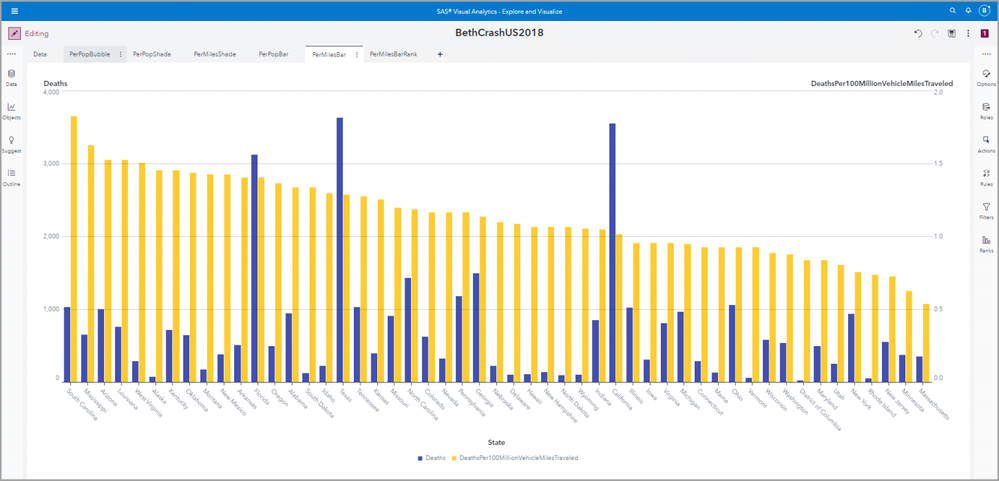We can use the ranks feature in Visual Analytics to look at the top five states for number of accident fatalities and the top five states for accident fatality rate per 100 million miles driven.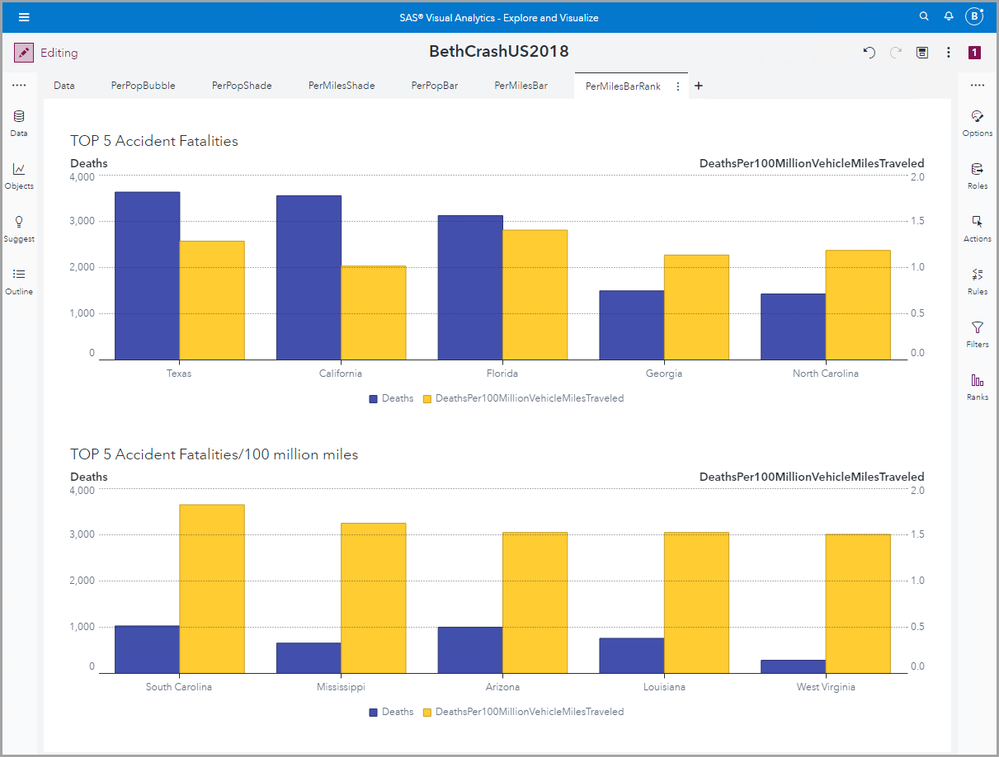### Using SAS to Rescale/Transform Data

You can rescale or transform your data in many SAS products. There are many ways to do this; I’ll just mention a couple examples in:

• SAS Studio
• Visual Analytics
• VDMML
• Enterprise Miner

In SAS Studio you can code or use tasks. Examples of simple code to transform variables would be something like:

SqrtX=sqrt(x);
Log10X=log10(x);
InverseSqrtX=-1/sqrt(x);

PROC STDIZE provides 18 standardization methods. It allows you to standardize variables by subtracting a location measure and dividing by a scale measure to, for example, get a z-score with a mean of 0 and a standard deviation of 1.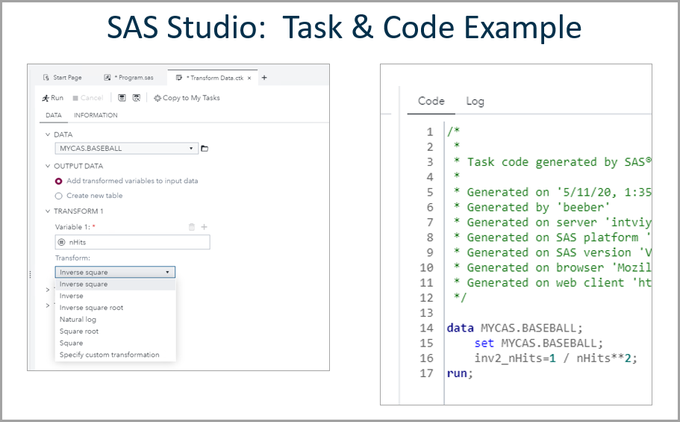In SAS Visual Analytics, you can use Calculated Items to transform variables.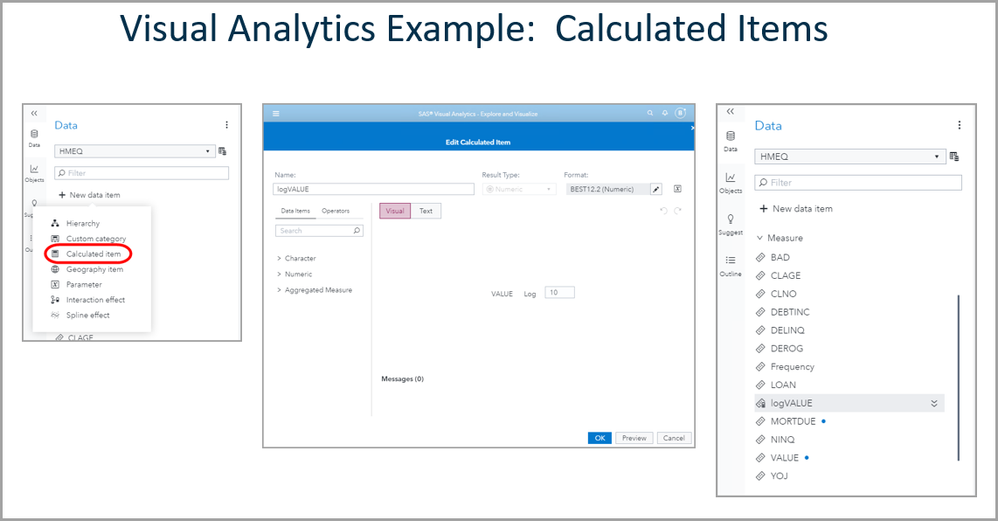With SAS Visual Data Mining and Machine Learning’s processImages action, you can, for example, take the derivative of an image.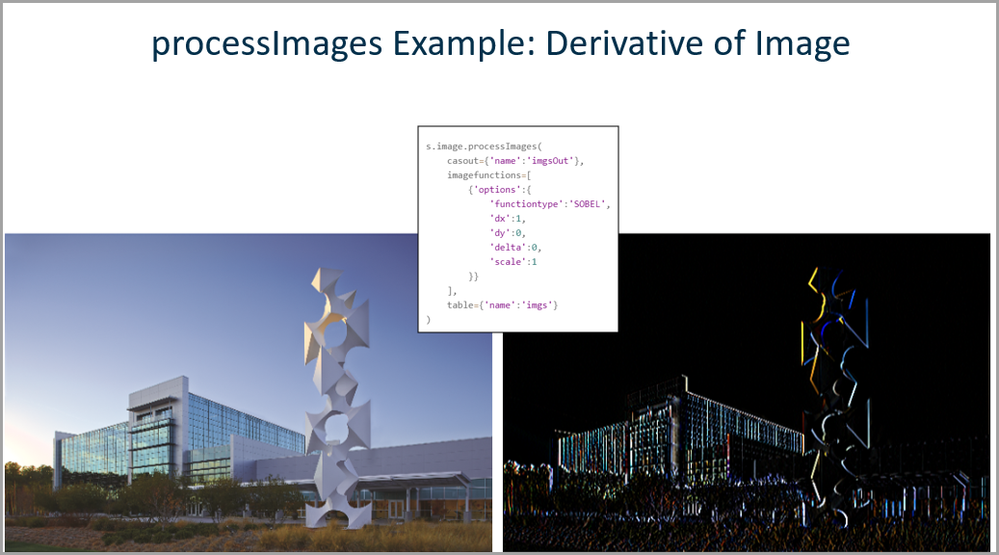In SAS Enterprise Miner, you can use the Transform Variables node.### Selecting Units and Methods

You usually want to try to use the units and methods accepted in your field. For example,

• Transportation
• Fatalities per 100,000 people
• Fatalities per million vehicle miles traveled
• Epidemiology
• Prevalence – number of existing cases / population total
• Incidence – number of new cases per 100,000 people at risk per year (e.g., for ovarian cancer people at risk would be total number of women)
• Mortality Rate – number of deaths due to a disease divided by the total population

For example, we see in the SAS Visual Analytics 8.5 coronavirus dashboard below, COVID-19 prevalence reported by date for five countries.ASIDE:  Be aware that sometimes units are themselves logarithmic scales (such as decibels, pH and the Richter scale)